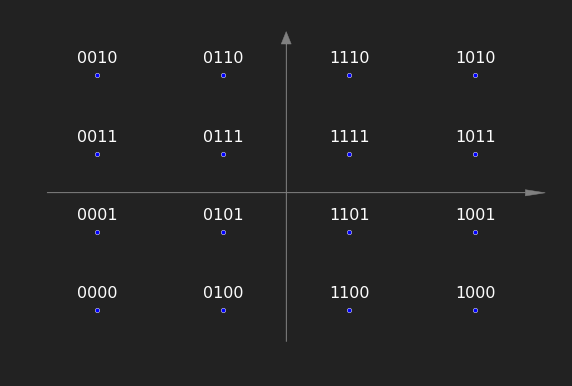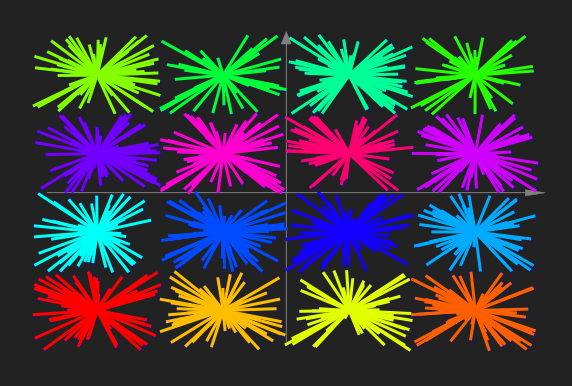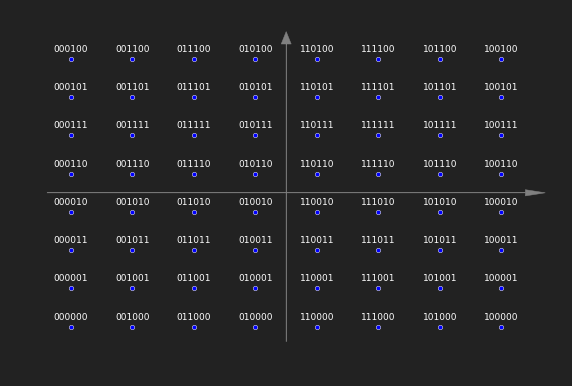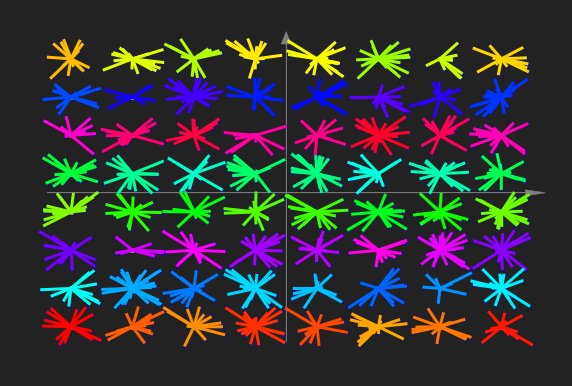I’m currently working on major updates to my GNU Radio WiFi Module. The goal is to make the module easier to extend with new algorithms, to make it more GNU Radio’ish and, finally, to get rid of the IT++ library.

As part of these updates I wanted to switch from demodulation with IT++ to GNU Radio’s constellation objects. Since there is no 64-QAM in GNU Radio and since the 16-QAM definition in gr-digital doesn’t match with what is defined for WiFi, I created my own constellations.

Turns out they are really cool and straightforward to use. In C++, I only had to define the complex constellation points and a decision maker that maps noisy samples to these constellation points. The whole magic for QPSK, for example, is something like:

constellation_qpsk_impl::constellation_qpsk_impl() {
const float level = sqrt(float(0.5));
d_constellation.resize(4);

d_constellation = gr_complex(-level, -level);
d_constellation = gr_complex( level, -level);
d_constellation = gr_complex(-level,  level);
d_constellation = gr_complex( level,  level);

d_rotational_symmetry = 4;
d_dimensionality = 1;
calc_arity();
}

unsigned int
constellation_qpsk_impl::decision_maker(const gr_complex *sample) {
return 2*(imag(*sample)>0) + (real(*sample)>0);
}


The tricky part is defining the SWIG bindings to make the constellations available in Python. I’m absolutely no SWIG expert, but I had the feeling that I had to work around some bugs or at least oddities of SWIG.

Obviously, I had to include the header that defines the abstract base class of constellation objects from gr-digital. The same header file, however, also contains the definitions of GNU Radio’s version of BPSK, QPSK, and 16-QAM. Actually, that shouldn’t be much of a problem since they are in different namespaces, but SWIG seems to mess that up.

So the first oddity is the need to explicitly ignore GNU Radio’s constellations when including the headers.

%ignore gr::digital::constellation_bpsk;
%ignore gr::digital::constellation_qpsk;
%ignore gr::digital::constellation_16qam;


With this change, I came one step further, but for whatever reason SWIG still messed up the namespaces of GNU Radio’s smart pointers. These pointers are used as proxies for the actual constructors so that you can’t instantiate the objects directly, but smart pointers of the objects. While it shouldn’t be needed to explicitly state the namespace when coding in a namespace, SWIG messed up the data types when not doing so. Again for QPSK, that meant that I had to do something like:

class IEEE802_11_API constellation_qpsk : virtual public digital::constellation
{
public:
typedef boost::shared_ptr<gr::ieee802_11::constellation_qpsk> sptr;
static sptr make();

protected:
constellation_qpsk();
};


With these changes it finally worked and I’m able to access the constellation objects also in Python and, for example, make some simple unit tests to compare the constellations to the ones given in the standard and to see whether the decision makers work.

In :
%matplotlib inline
import sys
import numpy as np
import matplotlib as mpl
import matplotlib.pyplot as plt
plt.style.use('bmh')
plt.rcParams['figure.facecolor'] = '#222222'
plt.rcParams['axes.facecolor'] = 'None'
plt.rcParams['figure.figsize'] = (10, 10.0*2/3)
plt.rcParams['font.size'] = 16
plt.rcParams['lines.linewidth'] = '3'
plt.rcParams['text.color'] = 'white'

import ieee802_11

def const_plot():
plt.axis('off')
plt.gca().get_xaxis().set_visible(False)
plt.gca().get_yaxis().set_visible(False)
plt.xlim(-1.4, 1.4)
plt.ylim(-1.5, 1.5)

###############################################
#                 16-QAM
###############################################

c = ieee802_11.constellation_16qam()
a = np.array(c.points())
p = np.average(np.abs(a)**2)
level = .1**.5

print "16-QAM, average power: {0:.4f}".format(p)

plt.scatter(a.real, a.imag)
const_plot()
for i, x in enumerate(a):
s = "{0:04b}".format(i)[::-1]
plt.text(x.real, x.imag+0.1, s, ha='center')

16-QAM, average power: 1.0000In :
#######################
# test decison maker
#######################

N = 1000
data = np.random.randint(0, 16, N)
orig_const = a[data]
noisy_const = orig_const + np.random.sample(N) * 2 * level - level +\
np.random.sample(N) * 2j * level - level * 1j

rx = np.array(map(lambda x: c.decision_maker_v([x]), noisy_const))
rx_const = a[rx]

if any(rx != data):
print "16-QAM: data does not match."
else:
print "16-QAM: points decoded successfully."

plt.scatter(a.real, a.imag)
plt.scatter(noisy_const.real, noisy_const.imag, marker='x')
const_plot()
for d, x, y in zip(rx, rx_const, noisy_const):
plt.plot([x.real, y.real], [x.imag, y.imag], color=mpl.cm.hsv(d/16.0))

16-QAM: points decoded successfully.In :
###############################################
#                 64-QAM
###############################################

c = ieee802_11.constellation_64qam()
a = np.array(c.points())
p = np.average(np.abs(a)**2)
level = (1.0/42)**.5

print "64-QAM, average power: {0:.4f}".format(p)

plt.scatter(a.real, a.imag)
const_plot()
for i, x in enumerate(a):
s = "{0:06b}".format(i)[::-1]
plt.text(x.real, x.imag+0.06, s, ha='center', fontsize=9)

64-QAM, average power: 1.0000In :
#######################
# test decison maker
#######################

N = 1000
data = np.random.randint(0, 64, N)
orig_const = a[data]
noisy_const = orig_const + np.random.sample(N) * 2 * level - level +\
np.random.sample(N) * 2j * level - level * 1j

rx = np.array(map(lambda x: c.decision_maker_v([x]), noisy_const))
rx_const = a[rx]

if any(rx != data):
print "64-QAM: data does not match."
else:
print "64-QAM: points decoded successfully."

plt.scatter(a.real, a.imag)
plt.scatter(noisy_const.real, noisy_const.imag, marker='x')
const_plot()
for d, x, y in zip(rx, rx_const, noisy_const):
plt.plot([x.real, y.real], [x.imag, y.imag], color=mpl.cm.hsv(d/64.0))

64-QAM: points decoded successfully.